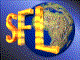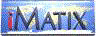| iMatix home page | << | < | > | >>SFLVersion 2.11

### sym_merge_tables

```#include "sflsymb.h"
int
sym_merge_tables (
SYMTAB *table,                      /*  Symbol table to import into      */
const SYMTAB *import)               /*  Symbol table to import from      */
```

#### Synopsis

Imports the contents of one symbol table into another. Will overwrite symbols with the same name. Returns the number of symbols imported. If there is a lack of available memory, will stop importing, and return negative the number of symbols imported so far.

#### Source Code - (sflsymb.c)

```{
SYMBOL
*symbol;                        /*  Next symbol in table             */
int
count = 0;

ASSERT (table);
ASSERT (import);

for (symbol = import-> symbols; symbol; symbol = symbol-> next)
{
if (sym assume symbol (table, symbol-> name, symbol-> value) == NULL)
{
return (-(count));            /*  Failed => negative value returned*/
}

count++;
}

return (count);                     /*  Success => postive value returned*/
}
```

 | << | < | > | >>Copyright © 1996-2000 iMatix Corporation